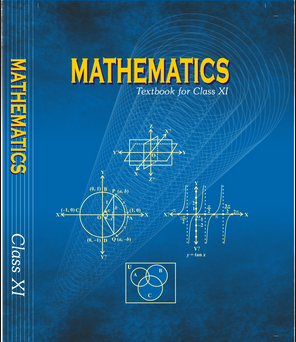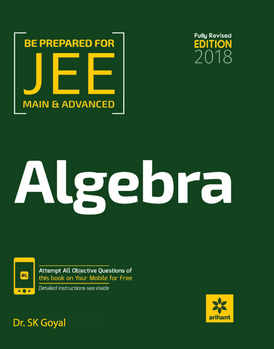# Mathematical Induction   Share

## What is Mathematical Induction

Mathematical Induction is a special technique used to prove a given statement about any well-ordered set n of natural numbers or we can say if a statement is true for n=1 and n=n than it always true for n=n+1. Mathematical Induction is a magic trick for defining additive, subtracting, multiplication and division properties of natural numbers. Directly, every year you will get 1 - 2 questions in JEE Main exam as well as in other engineering entrance exams. Indirectly, the concept of Mathematical Induction is widely used in many topics like Sequence and Series, Binomial Theorem, Trigonometry, etc.

This chapter checks your observing power, mathematical reasoning, and creativity towards the problem. In simple words, if you are preparing for JEE Mains or other engineering exams this chapter helps you to increase your calculative approach as well as thinking ability.  As you start doing problems on mathematical induction definitely your attitude becomes change toward mathematics.

## Why Mathematical Induction

Let us discuss your present situation,

1. If you work hard on your subjects than surely you will crack your dream exam.
2. Get selected in a good college.
3. Taught by good teachers.
4. Placed in a good company.
5. Live a good life.

If you are selected in a good college it proofs that you worked hard during their studies and vice versa. Here the second statement proofs their previous statement.

Let's take another example

Suppose that bicycles are parked in series and you pushed a cycle over another cycle what happened?

The first bicycle falls on adjacent cycle and this will continue till the last cycle will fall

Prepare Online for JEE Main/NEET

Crack JEE 2021 with JEE/NEET Online Preparation Program

Why this is happening have you ever think?

The reason comes from Mathematical Induction.

Now Solve some previous JEE Mains examples using Mathematical Induction:

From Trigonometry,

Let

on rationalizing,

Now if you put A=30º in the options you can easily find that option (b) is satisfied.

Now, you can check the given by using different angles but be careful with the values which you are choosing and check the solution at least for two values.

Similarly, In Sequence and Series,

We can consider any A.P. series like 1,3,5...... (where a=1, d=2)

G.P. series like 1,3,9.......(a=1, r=3)

H.P. series like

## Overview of the Mathematical Induction

The Principle of Mathematical Induction

The Principle of Mathematical Induction is defined as if a statement S(n) involving n natural number is true for n=1 and if S(n) is true for n=k (where k is any positive integer ) and it also proves that it is true for n=k+1 than S(n) is always true for natural numbers n.

## How to Prepare Mathematical Induction

Follow these tips to prepare the chapter Mathematical Induction.

1. Always check the given statement that it is true for n=1.
2. Analyze the statement given in the problem and try to convert it into the simplest form or standard form.
3. If you are taking any common from the solution be sure that it is correct.
4. Mathematical operation done in any stage of the solution should be as per the BODMAS rule.
5. Always in touch with the standard statement like the sum of n natural numbers, sum of n2, sum of n3, etc.

## Best Books For Preparation of Mathematical Induction:-

First, finish all the concepts, example and questions given in NCERT Maths Book. You must thorough with the theory of NCERT. Then you can refer to the book Cengage Mathematics Algebra. Mathematical Induction is explained very well in this book and there are ample amount of questions with crystal clear concepts. You can also refer to the book Arihant Algebra by SK Goyal or RD Sharma. But again the choice of reference book depends on person to person, find the book that best suits you the best depending on how well you are clear with the concepts and the difficulty of the questions you require.

## Maths Chapter-wise Notes for Engineering exams

 Chapters Chapters Name Chapter 1 Sets, Relations, and Functions Chapter 2 Complex Numbers and Quadratic Equations Chapter 3 Matrices and Determinants Chapter 4 Permutations and Combinations Chapter 5 Binomial Theorem and its Simple Applications Chapter 6 Sequence and Series Chapter 7 Limit, Continuity, and Differentiability Chapter 8 Integral Calculus Chapter 9 Differential Equations Chapter 10 Coordinate Geometry Chapter 11 Three Dimensional Geometry Chapter 12 Vector Algebra Chapter 13 Statistics and Probability Chapter 14 Trigonometry Chapter 15 Mathematical Reasoning

### Topics from Mathematical Induction

• Principle Of Mathematical Induction And Its Simple Applications ( JEE Main, COMEDK UGET ) (10 concepts)
• Principle of Mathematical Induction ( JEE Main, COMEDK UGET ) (2 concepts)

### Important Books for Mathematical Induction

••Exams
Articles
Questions